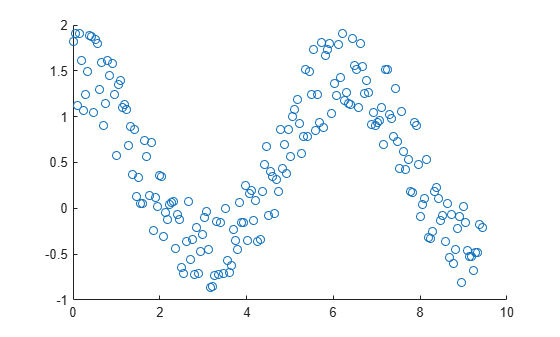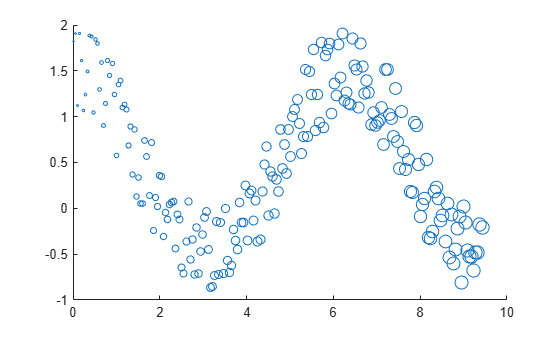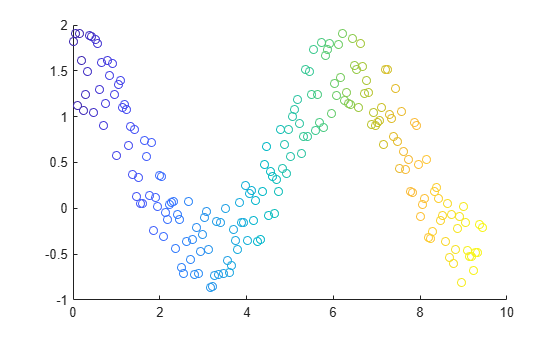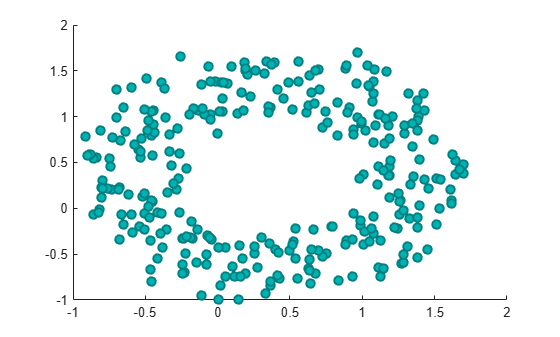Documentation

### This is machine translation

Mouseover text to see original. Click the button below to return to the English version of the page.

Note: This page has been translated by MathWorks. Click here to see
To view all translated materials including this page, select Country from the country navigator on the bottom of this page.

# scatter

## Syntax

``scatter(x,y)``
``scatter(x,y,sz)``
``scatter(x,y,sz,c)``
``scatter(___,'filled')``
``scatter(___,mkr)``
``scatter(___,Name,Value)``
``scatter(ax,___)``
``s = scatter(___)``

## Description

example

````scatter(x,y)` creates a scatter plot with circles at the locations specified by the vectors `x` and `y`. This type of graph is also known as a bubble plot.```

example

````scatter(x,y,sz)` specifies the circle sizes. To plot each circle with equal size, specify `sz` as a scalar. To plot each circle with a different size, specify `sz` as a vector with length equal to the length of `x` and `y`.```

example

````scatter(x,y,sz,c)` specifies the circle colors. To plot all circles with the same color, specify `c` as a color name or an RGB triplet. To use varying color, specify `c` as a vector or a three-column matrix of RGB triplets.```

example

````scatter(___,'filled')` fills in the circles. Use the `'filled'` option with any of the input argument combinations in the previous syntaxes.```

example

````scatter(___,mkr)` specifies the marker type.```

example

````scatter(___,Name,Value)` modifies the scatter chart using one or more name-value pair arguments. For example, `'LineWidth',2` sets the marker outline width to 2 points.```

example

````scatter(ax,___)` plots into the axes specified by `ax` instead of into the current axes. The option `ax` can precede any of the input argument combinations in the previous syntaxes.```

example

````s = scatter(___)` returns the `Scatter` object. Use `s` to make future modifications to the scatter chart after it is created.```

## Examples

collapse all

Create `x` as 200 equally spaced values between 0 and $3\pi$. Create `y` as cosine values with random noise. Then, create a scatter plot.

```x = linspace(0,3*pi,200); y = cos(x) + rand(1,200); scatter(x,y)```Create a scatter plot using circles with different sizes. Specify the size in points squared

```x = linspace(0,3*pi,200); y = cos(x) + rand(1,200); sz = linspace(1,100,200); scatter(x,y,sz)```Corresponding elements in `x`, `y`, and `sz` determine the location and size of each circle. To plot all circles with the equal area, specify `sz` as a numeric scalar.

Create a scatter plot and vary the circle color.

```x = linspace(0,3*pi,200); y = cos(x) + rand(1,200); c = linspace(1,10,length(x)); scatter(x,y,[],c)```Corresponding elements in `x`, `y`, and `c` determine the location and color of each circle. The `scatter` function maps the elements in `c` to colors in the current colormap.

Create a scatter plot and fill in the markers. `scatter` fills each marker using the color of the marker edge.

```x = linspace(0,3*pi,200); y = cos(x) + rand(1,200); sz = 25; c = linspace(1,10,length(x)); scatter(x,y,sz,c,'filled')```Create vectors `x` and `y` as sine and cosine values with random noise. Then, create a scatter plot and use diamond markers with an area of 140 points squared.

```theta = linspace(0,2*pi,150); x = sin(theta) + 0.75*rand(1,150); y = cos(theta) + 0.75*rand(1,150); sz = 140; scatter(x,y,sz,'d')```Create vectors `x` and `y` as sine and cosine values with random noise. Create a scatter plot and set the marker edge color, marker face color, and line width.

```theta = linspace(0,2*pi,300); x = sin(theta) + 0.75*rand(1,300); y = cos(theta) + 0.75*rand(1,300); sz = 40; scatter(x,y,sz,'MarkerEdgeColor',[0 .5 .5],... 'MarkerFaceColor',[0 .7 .7],... 'LineWidth',1.5)```Create a figure with two subplots and add a scatter plot to each subplot. Use filled diamond markers for the scatter plot in the lower subplot.

```x = linspace(0,3*pi,200); y = cos(x) + rand(1,200); ax1 = subplot(2,1,1); scatter(ax1,x,y) ax2 = subplot(2,1,2); scatter(ax2,x,y,'filled','d')```Create a scatter plot and return the scatter series object, `s`.

```theta = linspace(0,1,500); x = exp(theta).*sin(100*theta); y = exp(theta).*cos(100*theta); s = scatter(x,y);```Use `s` to query and set properties of the scatter series after it has been created. Set the line width to `0.6` point. Set the marker edge color to blue. Set the marker face color using an RGB triplet color.

Note: Starting in R2014b, you can use dot notation to set properties. If you are using an earlier release, use the `set` function instead, such as `set(s,'LineWidth',0.6)`.

```s.LineWidth = 0.6; s.MarkerEdgeColor = 'b'; s.MarkerFaceColor = [0 0.5 0.5];```## Input Arguments

collapse all

x values, specified as a vector. `x` and `y` must be vectors of equal length.

Data Types: `single` | `double` | `int8` | `int16` | `int32` | `int64` | `uint8` | `uint16` | `uint32` | `uint64` | `categorical` | `datetime` | `duration`

y values, specified as a vector. `x` and `y` must be vectors of equal length.

Data Types: `single` | `double` | `int8` | `int16` | `int32` | `int64` | `uint8` | `uint16` | `uint32` | `uint64` | `categorical` | `datetime` | `duration`

Marker area in points squared, specified in one of these forms:

• Numeric scalar — Plot all markers with equal size.

• Row or column vector — Use different sizes for each marker. Corresponding elements in `x`, `y`, and `sz` determine the location and area of each marker. The length of `sz` must equal the length of `x` and `y`.

• `[]` — Use the default area of 36 points squared.

The units for the marker area is points squared.

Example: `50`

Example: ```[36 25 25 17 46]```

Marker color, specified in one of these forms:

• RGB triplet or color name — Plot all markers with the same color.

• Three column matrix of RGB triplets — Use different colors for each marker. Each row of the matrix specifies an RGB triplet color for the corresponding marker. The number of rows must equal the length of `x` and `y`.

• Vector — Use different colors for each marker and linearly map values in `c` to the colors in the current colormap. The length of `c` must equal the length of `x` and `y`. To change the colormap for the axes, use the `colormap` function.

If you have three points in the scatter plot and want the colors to be indices into the colormap, specify `c` as a three-element column vector.

An RGB triplet is a three-element row vector whose elements specify the intensities of the red, green, and blue components of the color. The intensities must be in the range `[0,1]`; for example, `[0.4 0.6 0.7]`. Alternatively, you can specify some common colors by name. This table lists the long and short color name options and the equivalent RGB triplet values.

OptionDescriptionEquivalent RGB Triplet
`'red'` or `'r'`Red`[1 0 0]`
`'green'` or `'g'`Green`[0 1 0]`
`'blue'` or `'b'`Blue`[0 0 1]`
`'yellow'` or `'y'`Yellow`[1 1 0]`
`'magenta'` or `'m'`Magenta`[1 0 1]`
`'cyan'` or `'c'`Cyan`[0 1 1]`
`'white'` or `'w'`White`[1 1 1]`
`'black'` or `'k'`Black`[0 0 0]`

Example: `'k'`

Example: ```[1 2 3 4]```

Marker type, specified as one of the values listed in this table.

ValueDescription
`'o'`Circle
`'+'`Plus sign
`'*'`Asterisk
`'.'`Point
`'x'`Cross
`'square'` or `'s'`Square
`'diamond'` or `'d'`Diamond
`'^'`Upward-pointing triangle
`'v'`Downward-pointing triangle
`'>'`Right-pointing triangle
`'<'`Left-pointing triangle
`'pentagram'` or `'p'`Five-pointed star (pentagram)
`'hexagram'` or `'h'`Six-pointed star (hexagram)
`'none'`No markers

Option to fill the interior of the markers, specified as `'filled'`. Use this option with markers that have a face, for example, `'o'` or `'square'`. Markers that do not have a face and contain only edges do not draw (`'+'`, `'*'`, `'.'`, and `'x'`).

The `'filled'` option sets the `MarkerFaceColor` property of the `Scatter` object to `'flat'` and the `MarkerEdgeColor` property to `'none'`, so the marker faces draw, but the edges do not.

Target axes, specified as an `Axes` object, a `PolarAxes` object, or a `GeographicAxes` object. If you do not specify the axes and if the current axes are Cartesian axes, then the `scatter` function uses the current axes. To plot into polar axes, specify the `PolarAxes` object as the first input argument or use the `polarscatter` function. To plot into geographic axes, specify the `GeographicAxes` object as the first input argument or use the `geoscatter` function.

### Name-Value Pair Arguments

Specify optional comma-separated pairs of `Name,Value` arguments. `Name` is the argument name and `Value` is the corresponding value. `Name` must appear inside quotes. You can specify several name and value pair arguments in any order as `Name1,Value1,...,NameN,ValueN`.

Example: `'MarkerFaceColor','red'` sets the marker face color to red.

The `Scatter` object properties listed here are only a subset. For a complete list, see Scatter Properties.

Marker outline color, specified `'flat'`, an RGB triplet, a hexadecimal color code, a color name, or a short name. The default value of `'flat'` uses colors from the `CData` property.

For a custom color, specify an RGB triplet or a hexadecimal color code.

• An RGB triplet is a three-element row vector whose elements specify the intensities of the red, green, and blue components of the color. The intensities must be in the range `[0,1]`; for example, ```[0.4 0.6 0.7]```.

• A hexadecimal color code is a character vector or a string scalar that starts with a hash symbol (`#`) followed by three or six hexadecimal digits, which can range from `0` to `F`. The values are not case sensitive. Thus, the color codes `'#FF8800'`, `'#ff8800'`, `'#F80'`, and `'#f80'` are equivalent.

Alternatively, you can specify some common colors by name. This table lists the named color options, the equivalent RGB triplets, and hexadecimal color codes.

Color NameShort NameRGB TripletHexadecimal Color CodeAppearance
`'red'``'r'``[1 0 0]``'#FF0000'``'green'``'g'``[0 1 0]``'#00FF00'``'blue'``'b'``[0 0 1]``'#0000FF'``'cyan'` `'c'``[0 1 1]``'#00FFFF'``'magenta'``'m'``[1 0 1]``'#FF00FF'``'yellow'``'y'``[1 1 0]``'#FFFF00'``'black'``'k'``[0 0 0]``'#000000'``'white'``'w'``[1 1 1]``'#FFFFFF'``'none'`Not applicableNot applicableNot applicableNo color

Here are the RGB triplets and hexadecimal color codes for the default colors MATLAB® uses in many types of plots.

RGB TripletHexadecimal Color CodeAppearance
`[0 0.4470 0.7410]``'#0072BD'``[0.8500 0.3250 0.0980]``'#D95319'``[0.9290 0.6940 0.1250]``'#EDB120'``[0.4940 0.1840 0.5560]``'#7E2F8E'``[0.4660 0.6740 0.1880]``'#77AC30'``[0.3010 0.7450 0.9330]``'#4DBEEE'``[0.6350 0.0780 0.1840]``'#A2142F'`Example: `[0.5 0.5 0.5]`

Example: `'blue'`

Example: `'#D2F9A7'`

Marker fill color, specified as `'flat'`, `'auto'`, an RGB triplet, a hexadecimal color code, a color name, or a short name. The `'flat'` option uses the `CData` values. The `'auto'` option uses the same color as the `Color` property for the axes.

For a custom color, specify an RGB triplet or a hexadecimal color code.

• An RGB triplet is a three-element row vector whose elements specify the intensities of the red, green, and blue components of the color. The intensities must be in the range `[0,1]`; for example, ```[0.4 0.6 0.7]```.

• A hexadecimal color code is a character vector or a string scalar that starts with a hash symbol (`#`) followed by three or six hexadecimal digits, which can range from `0` to `F`. The values are not case sensitive. Thus, the color codes `'#FF8800'`, `'#ff8800'`, `'#F80'`, and `'#f80'` are equivalent.

Alternatively, you can specify some common colors by name. This table lists the named color options, the equivalent RGB triplets, and hexadecimal color codes.

Color NameShort NameRGB TripletHexadecimal Color CodeAppearance
`'red'``'r'``[1 0 0]``'#FF0000'``'green'``'g'``[0 1 0]``'#00FF00'``'blue'``'b'``[0 0 1]``'#0000FF'``'cyan'` `'c'``[0 1 1]``'#00FFFF'``'magenta'``'m'``[1 0 1]``'#FF00FF'``'yellow'``'y'``[1 1 0]``'#FFFF00'``'black'``'k'``[0 0 0]``'#000000'``'white'``'w'``[1 1 1]``'#FFFFFF'``'none'`Not applicableNot applicableNot applicableNo color

Here are the RGB triplets and hexadecimal color codes for the default colors MATLAB uses in many types of plots.

RGB TripletHexadecimal Color CodeAppearance
`[0 0.4470 0.7410]``'#0072BD'``[0.8500 0.3250 0.0980]``'#D95319'``[0.9290 0.6940 0.1250]``'#EDB120'``[0.4940 0.1840 0.5560]``'#7E2F8E'``[0.4660 0.6740 0.1880]``'#77AC30'``[0.3010 0.7450 0.9330]``'#4DBEEE'``[0.6350 0.0780 0.1840]``'#A2142F'`Example: `[0.3 0.2 0.1]`

Example: `'green'`

Example: `'#D2F9A7'`

Width of marker edge, specified as a positive value in point units.

Example: `0.75`

## Output Arguments

collapse all

`Scatter` object. Use `s` to access and modify properties of the scatter chart after it has been created.

Download ebook